Thermodynamic Potential MCQ Level – 1

# Thermodynamic Potential MCQ Level – 1

Test Description

## 10 Questions MCQ Test Topic wise Tests for IIT JAM Physics | Thermodynamic Potential MCQ Level – 1

Thermodynamic Potential MCQ Level – 1 for IIT JAM 2022 is part of Topic wise Tests for IIT JAM Physics preparation. The Thermodynamic Potential MCQ Level – 1 questions and answers have been prepared according to the IIT JAM exam syllabus.The Thermodynamic Potential MCQ Level – 1 MCQs are made for IIT JAM 2022 Exam. Find important definitions, questions, notes, meanings, examples, exercises, MCQs and online tests for Thermodynamic Potential MCQ Level – 1 below.
Solutions of Thermodynamic Potential MCQ Level – 1 questions in English are available as part of our Topic wise Tests for IIT JAM Physics for IIT JAM & Thermodynamic Potential MCQ Level – 1 solutions in Hindi for Topic wise Tests for IIT JAM Physics course. Download more important topics, notes, lectures and mock test series for IIT JAM Exam by signing up for free. Attempt Thermodynamic Potential MCQ Level – 1 | 10 questions in 30 minutes | Mock test for IIT JAM preparation | Free important questions MCQ to study Topic wise Tests for IIT JAM Physics for IIT JAM Exam | Download free PDF with solutions
 1 Crore+ students have signed up on EduRev. Have you?
Thermodynamic Potential MCQ Level – 1 - Question 1

### The thermodynamic relation expressing the change at temperature with volume at constant entropy is Select one:

Detailed Solution for Thermodynamic Potential MCQ Level – 1 - Question 1

From thermodynamic Maxwell equation :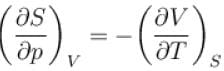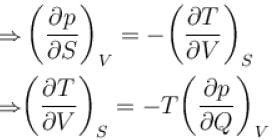The correct answer is: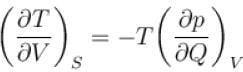Thermodynamic Potential MCQ Level – 1 - Question 2

### A sample of an ideal gas with initial pressure p and volume V is taken through an isothermal expansion proceed during which the change in entropy is found to be ΔS. The universal gas constant is R. Then, the work done by the gas is given by Select one:

Detailed Solution for Thermodynamic Potential MCQ Level – 1 - Question 2

By ideal gas equal,
pV = nRT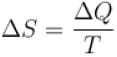Multiplying both the equations,
pVΔS = nRΔQ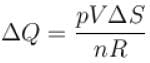∴ For isothermal expansion, ΔT = 0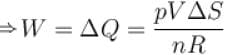The correct answer is: pVΔS\nR

Thermodynamic Potential MCQ Level – 1 - Question 3

### The Gibb’s potential is defined as : Select one:

Detailed Solution for Thermodynamic Potential MCQ Level – 1 - Question 3

Gibb’s free energy, denoted by G, combines enthalpy and entropy into a single value
The free energy is defined as follows
G = H – TS
Since H = U + pV
G = U + pV – TS
The correct answer is:
G = U + pV – TS

Thermodynamic Potential MCQ Level – 1 - Question 4

The thermodynamical relation expressing TdS equation.
Select one:

Detailed Solution for Thermodynamic Potential MCQ Level – 1 - Question 4

Let the entropy S of a thermodynamic system be a function of temperature T and Volume V i.e.
S = f(T, V)
Since dS is perfect differential, we can write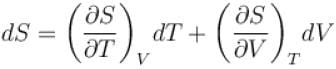Multiplying by T, we get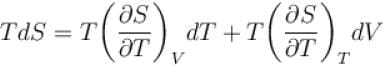For any substance, the specific heat at constant volume is given by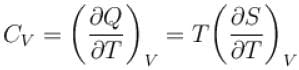Using dQ = TdS
Also, from Maxwell’s equation, we have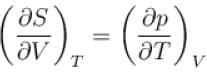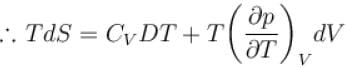The correct answer is: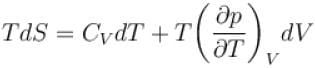Thermodynamic Potential MCQ Level – 1 - Question 5

The thermodynamical potential enthalpy H = U + pV, then which of the following relation hold true?
Select one:

Detailed Solution for Thermodynamic Potential MCQ Level – 1 - Question 5

H = U + pV
dH = dU + pdV + Vdp
dH = dQ + Vdp
⇒ dH = TdS + Vdp
Take S to be constant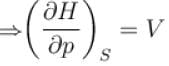take p to be constant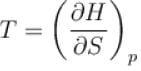The correct answer is: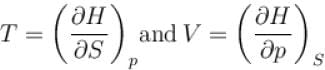Thermodynamic Potential MCQ Level – 1 - Question 6

The combined form of first and second law of thermodynamics is given by (p = pressure, V = volume, T = temperature, U = internal energy, S = entropy, Q = quantity of heat)
Select one:

Detailed Solution for Thermodynamic Potential MCQ Level – 1 - Question 6

According to first law of thermodynamics
dQ = dU + pdV ...(1)
According to second law of thermodynamics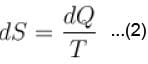Combined form of (1) and (2)
TdS = dU + pdV
The correct answer is: TdS = dU + pdV

Thermodynamic Potential MCQ Level – 1 - Question 7

Helmholtz free energy in given as F = U – Ts then which of the relations hold true?
Select one:

Detailed Solution for Thermodynamic Potential MCQ Level – 1 - Question 7

F = U – TS
dF = dU – TdS + SdT
take V to be constant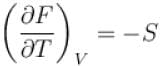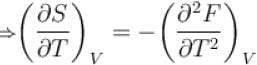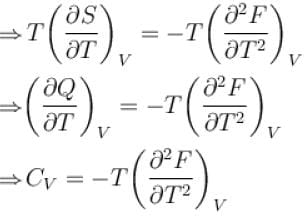The correct answer is: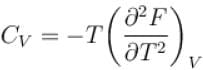Thermodynamic Potential MCQ Level – 1 - Question 8

The Gibb’s function G in thermodynamics is defined as
G = H – TS
In an isothermal, isobaric, reversible process, G
(where H = Enthalpy, T = Temperature, S = Entropy)
Select one:

Detailed Solution for Thermodynamic Potential MCQ Level – 1 - Question 8

Condition for a change to occur in any process
dU – TdS ≤ –pdV
d(U – TS + pV) ≤ 0
⇒ d(H – TS) ≤ 0
Since, G = H – TS
We get dG ≤ 0
it means in isothermal, isobaric and reversible process,
dG = 0 or G = constant
The correct answer is: Remains constant but not zero

Thermodynamic Potential MCQ Level – 1 - Question 9

Gibb’s thermodynamical potential can be represented as G = H – TS. Which relation hold true?
[Where H = Enthalpy, S = Entropy]
Select one:

Detailed Solution for Thermodynamic Potential MCQ Level – 1 - Question 9

We know that
dU = TdS – pdV + µdN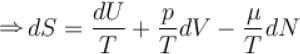take U to be constant and N to be constant
we get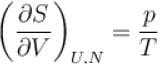The correct answer is: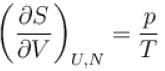Thermodynamic Potential MCQ Level – 1 - Question 10

The enthalpy of unit mass for any system is
Select one:

Detailed Solution for Thermodynamic Potential MCQ Level – 1 - Question 10

The enthalpy of a homogeneous system is defined as
H = U + pV
The U term can be interpreted as the energy required to create the system, and the pV terms as the energy that would be required to “make room” for the system if pressure of the environment remained constant.
The correct answer is:
H = U + pV

## Topic wise Tests for IIT JAM Physics

217 tests
 Use Code STAYHOME200 and get INR 200 additional OFF Use Coupon Code
Information about Thermodynamic Potential MCQ Level – 1 Page
In this test you can find the Exam questions for Thermodynamic Potential MCQ Level – 1 solved & explained in the simplest way possible. Besides giving Questions and answers for Thermodynamic Potential MCQ Level – 1, EduRev gives you an ample number of Online tests for practice

217 tests